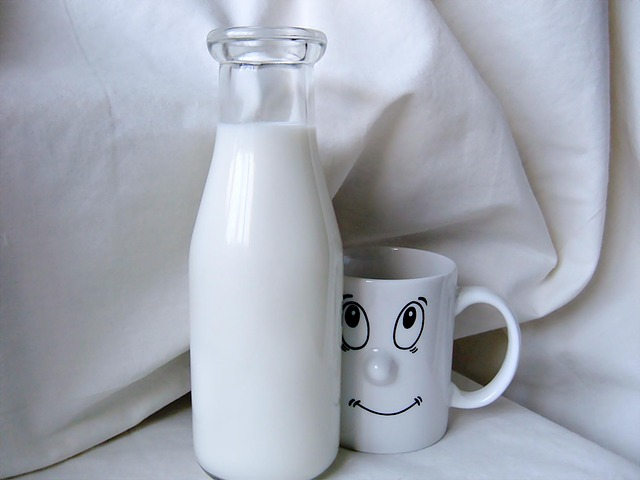The following are some of the foods which have a high amount of calcium. These values are provided by the US Department of Agriculture (USDA) these values of calcium are in 100 grams of the food.

Fishes (Cod Fish) = 11 mg

Beef = 12 mg

Rice = 19 mg

Orange juice = 40 mg

Lentils = 79 mg

Ricotta = 90 mg

Chickpeas = 53 mg

Bean curd = 114 mg

Hazelnuts = 114 mg

Boiled eggs = 50 mg

Spinach = 99 mg

Parmesan cheese = 1140 mg

Dried milk = 909 mg

Molasses = 273 mg

Collard (green) = 232 mg

Kale = 150 mg

Nonfat cow milk = 122 mg

Almonds = 234 mg

Cheddar cheese = 720 mg

How many valence electrons does calcium have?

The electronic configuration of Calcium is 1s2, 2s2, 2p6, 3s2, 3p6, 4s2. Calcium has two valence electrons. The total number of electrons in an electrically neutral calcium atom is 20.How much calcium in milk?

The calcium value is different in different milk. It is depending on the diet of the animal, place and type and breed of animal etc. The following are some of the value of calcium in different types of milk.

These values are recorded in 100 ml of milk.

Cow milk = 120 mg.

Water buffalo =195 mg.

Goat milk =100 mg

Sheep milk = 170 mg.

Fortified Soy, Oat and almond milk has the following value of calcium in 250 ml of milk.

Oat milk = 350 mg

Soy milk = 301 mg

Almond milk = 516 mg